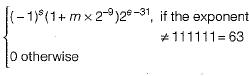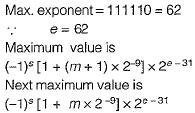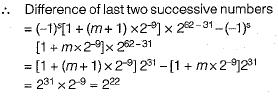Courses

# Floating Point Number (Basic Level)- 1

## 10 Questions MCQ Test Question Bank for GATE Computer Science Engineering | Floating Point Number (Basic Level)- 1

Description
This mock test of Floating Point Number (Basic Level)- 1 for Computer Science Engineering (CSE) helps you for every Computer Science Engineering (CSE) entrance exam. This contains 10 Multiple Choice Questions for Computer Science Engineering (CSE) Floating Point Number (Basic Level)- 1 (mcq) to study with solutions a complete question bank. The solved questions answers in this Floating Point Number (Basic Level)- 1 quiz give you a good mix of easy questions and tough questions. Computer Science Engineering (CSE) students definitely take this Floating Point Number (Basic Level)- 1 exercise for a better result in the exam. You can find other Floating Point Number (Basic Level)- 1 extra questions, long questions & short questions for Computer Science Engineering (CSE) on EduRev as well by searching above.
QUESTION: 1

### Booth's algorithm is used in floating-point

Solution:

Booth’s algorithm is used for floating point multiplication.

QUESTION: 2

### Booth’s coding in 8-bits for the decimal number - 57 is ________ .

Solution:

0 - 100 + 100 - 1 evaluates to 57 in Booth’s coding as below: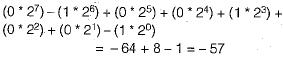QUESTION: 3

### Booth’s algorithm for integer multiplication gives worst performance when the multiplier pattern is

Solution:

Booth recording of a multiplier are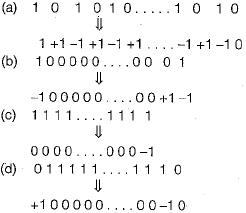Therefore, option (a) gives worst performance because it contains maximum number of 1 's.

QUESTION: 4

Consider the following floating point number representation.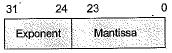The exponent is in 2’s complement representation and mantissa is in the sign-magnitude representation. The range of the magnitude of the normalized numbers in this representation is

Solution: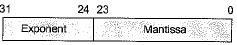The range of the magnitude of the normalized numbers is as follows: 0.5 to (1 - 2-23).

QUESTION: 5

Considerthe values A = 2.0 x 1030, B = -2.0x1030, C = 1.0 and the sequence. What will be the values of X and Y if the following sequence of statements are carried outSolution: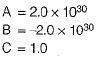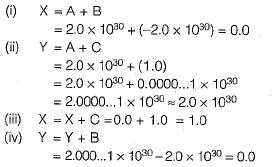Therefore, after the sequence value of X and Y are 1.0 and 0.0 respectively.

QUESTION: 6

The decimal value 0.25

Solution:

0.25 x 2 = 0.50;
0.50 x 2 = 1.00
Therefore, the decimal value 0.25 is equivalent to binary 0.01.

QUESTION: 7

The 2’s complement representation of the decimal value - 15 is

Solution:

The 2’s complement of negative numbers are represented as the binary number that when added to a positive number of the same magnitude equals zero
+15 = 0 1 1 1 1
The 2’s complement representation of the decimal value -15 is
-15 in 2’s complement = 10001

QUESTION: 8

Sign extension is the step in

Solution:

Sign extension is the operation, in computer arithmetic, of increasing the number of bits of a binary number while preserving the number’s sign (positive/negative) and value.

QUESTION: 9

Assuming all numbers are in 2’s complement representation, which of the following numbers is divisible by 11111011?

Solution: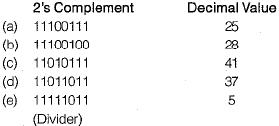So -25 is divisible by -5 so we can say 11100111 is divisible by 11111011.

QUESTION: 10

The following is a scheme for floating point number representation using 16 bits.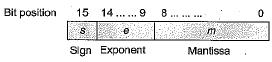Let s, e, and m be the numbers represented in binary in the sign, exponent, and mantissa fields respectively. Then the floating point number represented is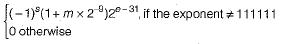What is the maximum difference between two successive real numbers representable in this system?

Solution: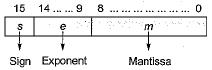Then the floating point number represented